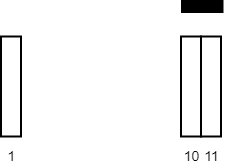##### Welcome to Subscribe On Youtube

Formatted question description: https://leetcode.ca/all/2271.html

# 2271. Maximum White Tiles Covered by a Carpet

• Difficulty: Medium.
• Related Topics: Array, Binary Search, Greedy, Sorting, Prefix Sum.
• Similar Questions: Maximum Number of Vowels in a Substring of Given Length.

## Problem

You are given a 2D integer array tiles where tiles[i] = [li, ri] represents that every tile j in the range li <= j <= ri is colored white.

You are also given an integer carpetLen, the length of a single carpet that can be placed anywhere.

Return the **maximum number of white tiles that can be covered by the carpet**.

Example 1:Input: tiles = [[1,5],[10,11],[12,18],[20,25],[30,32]], carpetLen = 10
Output: 9
Explanation: Place the carpet starting on tile 10.
It covers 9 white tiles, so we return 9.
Note that there may be other places where the carpet covers 9 white tiles.
It can be shown that the carpet cannot cover more than 9 white tiles.


Example 2:Input: tiles = [[10,11],[1,1]], carpetLen = 2
Output: 2
Explanation: Place the carpet starting on tile 10.
It covers 2 white tiles, so we return 2.


Constraints:

• 1 <= tiles.length <= 5 * 104

• tiles[i].length == 2

• 1 <= li <= ri <= 109

• 1 <= carpetLen <= 109

• The tiles are non-overlapping.

## Solution (Java, C++, Python)

• class Solution {
public int maximumWhiteTiles(int[][] tiles, int carpetLength) {
Arrays.sort(tiles, Comparator.comparingInt(x -> x));
int currentCover = Math.min(tiles - tiles + 1, carpetLength);
int maxCover = currentCover;
int tail = 0;
while (tail < tiles.length && head < tiles.length && maxCover < carpetLength) {
if (tiles[head] - tiles[tail] + 1 <= carpetLength) {
maxCover = Math.max(maxCover, currentCover);
} else {
maxCover = Math.max(maxCover, currentCover + possiblePartialCoverOverCurrentHead);
currentCover = currentCover - (tiles[tail] - tiles[tail] + 1);
++tail;
}
}
return maxCover;
}
}

• Todo

• class Solution:
def maximumWhiteTiles(self, tiles: List[List[int]], carpetLen: int) -> int:
tiles.sort()
n = len(tiles)
s = ans = j = 0
for i, (li, ri) in enumerate(tiles):
while j < n and tiles[j] - li + 1 <= carpetLen:
s += tiles[j] - tiles[j] + 1
j += 1
if j < n and li + carpetLen > tiles[j]:
ans = max(ans, s + li + carpetLen - tiles[j])
else:
ans = max(ans, s)
s -= ri - li + 1
return ans

############

# 2271. Maximum White Tiles Covered by a Carpet
# https://leetcode.com/problems/maximum-white-tiles-covered-by-a-carpet/

class Solution:
def maximumWhiteTiles(self, tiles: List[List[int]], carpetLen: int) -> int:
res = 0
tiles.sort()

prefix = 
for x, y in tiles:
prefix.append(prefix[-1] + y - x + 1)

startPos = [x for x, _ in tiles]

for i, (x, y) in enumerate(tiles):
if y - x + 1 >= carpetLen:
return carpetLen

endIndex = bisect.bisect(startPos, x + carpetLen - 1) - 1
ex, ey = tiles[endIndex]

extra = 0

if ey - x + 1 > carpetLen:
extra = ey - x - carpetLen + 1

res = max(res, prefix[endIndex + 1] - prefix[i] - extra)

return res



Explain:

nope.

Complexity:

• Time complexity : O(n).
• Space complexity : O(n).